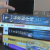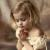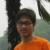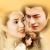## C++ 中两个类的互相引用导致 unresolved external symbol 的问题

guy_1 发布于 2016/06/16 09:25

```// Matrix4x4.h
#pragma once

namespace MiniMathLib
{
class Vector4;

class Matrix4x4
{
public:
Matrix4x4();
Matrix4x4(const Vector4 &r0, const Vector4 &r1, const Vector4      &r2, const Vector4 &r3);
Matrix4x4(const Matrix4x4 &m);

// 返回位于矩阵 r 行，c 列的元素
inline float operator()(int r, int c) const;
// 返回矩阵的第 r 行
inline Vector4 operator[](int r) const;

private:
float m;
};
}

// Vector4.h
#pragma once

namespace MiniMathLib
{
class Matrix4x4;

class Vector4
{
public:
float x, y, z, w;

Vector4();
Vector4(float x, float y, float z, float w);
Vector4(const Vector4 &v);

Vector4 operator+(const Vector4 &v) const;
Vector4 operator-(const Vector4 &v) const;
Vector4 operator*(float f) const;
Vector4 operator*(const Vector4 &v) const;
friend Vector4 operator*(float f, const Vector4 &v);
// 行向量与 4x4 矩阵的相乘
Vector4 operator*(const Matrix4x4 &m) const;
};
}

// Matrix4x4.cpp
#include "Matrix4x4.h"
#include "Vector4.h"

namespace MiniMathLib
{
// 省略一些函数定义

float Matrix4x4::operator()(int r, int c) const
{
return m[r][c];
}

float Matrix4x4::operator[](int r) const
{
return Vector4(m[r], m[r], m[r], m[r]);
}
}

// Vector4.cpp
#include "Vector4.h"
#include "Matrix4x4.h"

namespace MiniMathLib
{
// 省略一些函数定义

Vector4 Vector4::operator*(const Matrix4x4 &m) const
{
return x * m + y * m + z * m + w * m;
}
}```

`Vector4 operator*(const Matrix4x4 &m) const;`

```Vector4 Vector4::operator*(const Matrix4x4 &m) const
{
m(0, 0);
return Vector4();
}```

00inline Vector4 operator[](int r)const;0```class Vector4;  // 前置声明

// 返回矩阵的第 r 行
inline Vector4 operator[](int r) const;
// 上面返回的是一个Vector4对象，所以需要知道这个对象的内存布局，而前置声明不能做到。```

0#### 引用来自“乌合之众”的评论

```class Vector4;  // 前置声明

// 返回矩阵的第 r 行
inline Vector4 operator[](int r) const;
// 上面返回的是一个Vector4对象，所以需要知道这个对象的内存布局，而前置声明不能做到。```